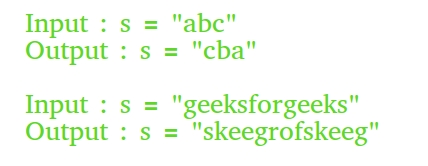# Different Methods to Reverse a String in C++

• Difficulty Level : Easy
• Last Updated : 18 May, 2022

The reversing of a string is nothing but simply substituting the last element of a string to the 1st position of the string.Different Methods to Reverse a String in C++ are:

• Making our own reverse function
• Using ‘inbuilt’ reverse function
• Using Constructor
• Using a temp file

### 1. Making a Custom Reverse Function For Swapping Characters

A. Using a first to last approach ‘for’ loop

## CPP

 `// C++ program to reverse a string  ` `// using first to last approach ` `// 'for' loop ` `#include ` `using` `namespace` `std; ` ` `  `// Function to reverse a string ` `void` `reverseStr(string& str) ` `{ ` `    ``int` `n = str.length(); ` ` `  `    ``// Swap character starting from two ` `    ``// corners ` `    ``for` `(``int` `i = 0; i < n / 2; i++) ` `        ``swap(str[i], str[n - i - 1]); ` `} ` ` `  `// Driver program ` `int` `main() ` `{ ` `    ``string str = ``"geeksforgeeks"``; ` `    ``reverseStr(str); ` `    ``cout << str; ` `    ``return` `0; ` `}`

Output :

`skeegrofskeeg`

B. Using a Last to First Approach ‘for‘ Loop

## C++

 `// C++ program to demonstrate reverse ` `// of a string using Last to First ` `// Approach 'for' Loop ` `#include ` `using` `namespace` `std; ` ` `  `// Function to reverse a string ` `void` `reverse(string str) ` `{ ` `    ``for` `(``int` `i = str.length() - 1; i >= 0; i--) ` `        ``cout << str[i]; ` `} ` ` `  `// Driver code ` `int` `main(``void``) ` `{ ` `    ``string s = ``"GeeksforGeeks"``; ` `    ``reverse(s); ` `    ``return` `(0); ` `}`

Output:

`skeegrofskeeG`### 2. Using the inbuilt “reverse” Function

There is a direct function in the “algorithm” header file for doing reverse that saves our time when programming.

```// Reverses elements in [begin, end]
void reverse (BidirectionalIterator begin,
BidirectionalIterator end);```

## CPP

 `// C++ program to illustrate the ` `// reversing of a string using  ` `// reverse() function ` `#include ` `using` `namespace` `std; ` `int` `main() ` `{ ` `    ``string str = ``"geeksforgeeks"``; ` ` `  `    ``// Reverse str[begin..end] ` `    ``reverse(str.begin(), str.end()); ` ` `  `    ``cout << str; ` `    ``return` `0; ` `}`

Output:

`skeegrofskeeg`

### 3. Reverse a String Using the Constructor

Passing reverse iterators to the constructor returns us a reversed string.

## CPP

 `// C++ program to reverse ` `// string using constructor ` `#include ` `using` `namespace` `std; ` `int` `main() ` `{ ` `    ``string str = ``"GeeksforGeeks"``; ` ` `  `    ``// Use of reverse iterators ` `    ``string rev = string(str.rbegin(), str.rend()); ` ` `  `    ``cout << rev << endl; ` `    ``return` `0; ` `}`

Output:

`skeeGrofskeeG`

## CPP

 `// C++ program to demonstrate ` `// reversing of string  ` `// using temporary string ` `#include ` `using` `namespace` `std; ` `int` `main() ` `{ ` ` `  `    ``string str = ``"GeeksforGeeks"``; ` `    ``int` `n = str.length(); ` `   `  `    ``// Temporary string to store the reverse ` `    ``string rev; ` `   `  `    ``for` `(``int` `i = n - 1; i >= 0; i--) ` `        ``rev.push_back(str[i]); ` ` `  `    ``cout << rev << endl; ` `    ``return` `0; ` `}`

Output:

`skeeGrofskeeG`

How could we get the reverse of a const string?

To get the reverse of a const string we have to first declare a ‘const string’ in a user-defined function following which we have declared then use the following algorithm for the calling of the desired objects.

`“const reverseConstString = function(string) { return string.split("").reverse().join("")” `

Example:

## C++

 `// C++ program to get reverse of a const string ` `#include ` `using` `namespace` `std; ` ` `  `// Function to reverse string and return ` `// reverse string pointer of that ` `char``* reverseConstString(``char` `const``* str) ` `{ ` `    ``// find length of string ` `    ``int` `n = ``strlen``(str); ` ` `  `    ``// create a dynamic pointer char array ` `    ``char``* rev = ``new` `char``[n + 1]; ` ` `  `    ``// copy of string to ptr array ` `    ``strcpy``(rev, str); ` ` `  `    ``// Swap character starting from two ` `    ``// corners ` `    ``for` `(``int` `i = 0, j = n - 1; i < j; i++, j--) ` `        ``swap(rev[i], rev[j]); ` ` `  `    ``// return pointer of the reversed string ` `    ``return` `rev; ` `} ` ` `  `// Driver code ` `int` `main(``void``) ` `{ ` `    ``const` `char``* s = ``"GeeksforGeeks"``; ` `    ``printf``(``"%s"``, reverseConstString(s)); ` `    ``return` `(0); ` `}`

Output:

`skeeGrofskeeG`